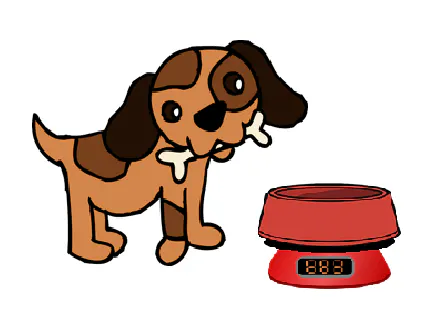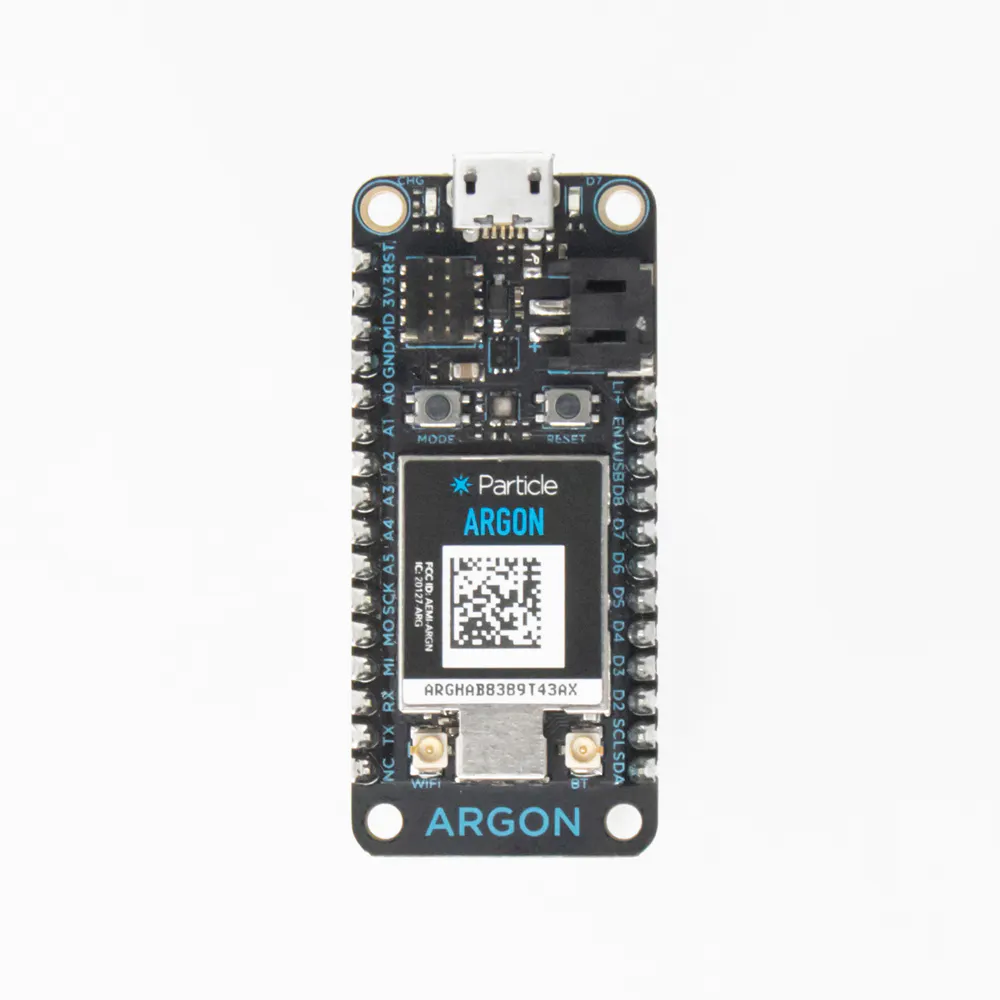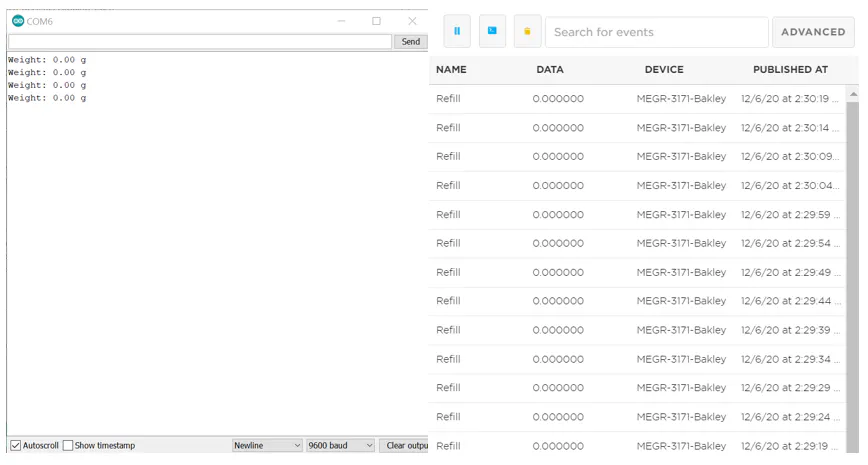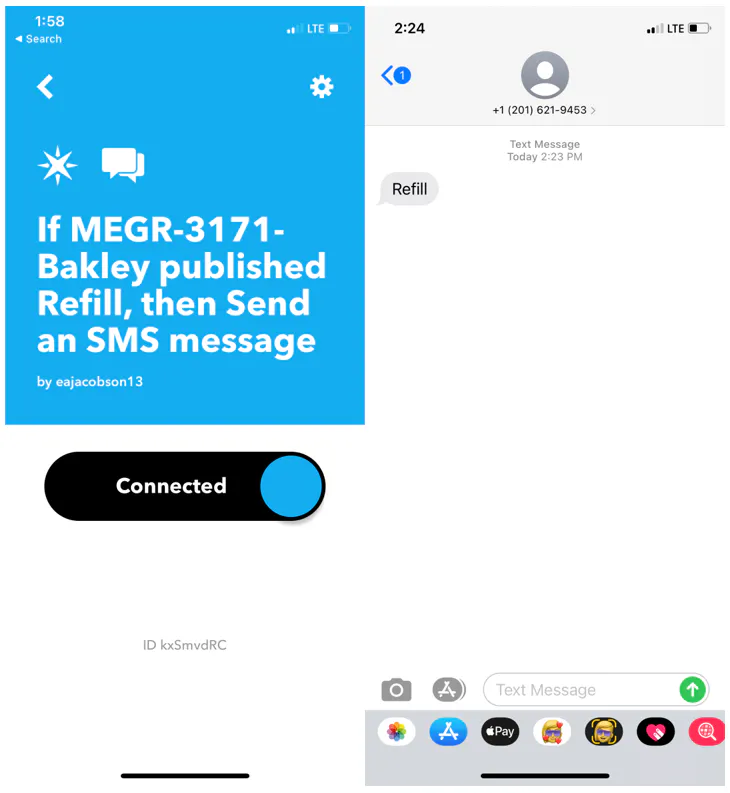Published

# Dog Bowl Capacity Tracker

It's very easy to forget to fill up your dog's bowl, so with this product you can get notified when the water bowl is running low!

BeginnerFull instructions provided210## Things used in this project

### Hardware components

×1Particle Argon
×2
 HX711 Amplifier
×1
 Jumper wires
×1

### Software apps and online servicesParticle Build Web IDE
 Particle CLIArduino IDEIFTTT Maker service

### Hand tools and fabrication machinesSoldering iron (generic)Solder Flux, Soldering

## Schematics

### Particle Events### IFTTT## Code

### Calibration

C/C++
```#include <LiquidCrystal_I2C_Spark.h>

#include "HX711.h"

#define DT  D1 //DT on HX711 amplifier connected to pin D1
#define SCK  D0 //SCK on HX711 amplifier connected to pin D0

HX711 scale(DT, SCK);

void setup() {
//blinks D7 LED to ensure code has started
pinMode(D7, OUTPUT);
digitalWrite(D7, HIGH);
delay(800);
digitalWrite(D7, LOW);

//starts and tares the scale
Serial.begin(9600);
scale.set_scale();
scale.tare();
}

void loop() {
float current_weight = scale.get_units(20);
float scale_factor = (current_weight/0.138); //finds the calibration using in kg by using a 138 g iphone
Serial.println(scale_factor); //displays the calibration factor in arduino IDE
delay(2000);
}
```

### Publish

C/C++
```#include <HX711ADC.h>
#include <LiquidCrystal_I2C_Spark.h>

#define DT  D1 //DT on HX711 amplifier connected to pin D1
#define SCK  D0 //SCK on HX711 amplifier connected to pin D0

float calibration_factor = 10000; //calibration factor found from "calibration.ino" code
float minimum_weight = 1.5; //set this to what weight (kg) on the scale when the dog bowl is low

void setup() {
//blinks D7 LED to ensure code has started
pinMode(D7, OUTPUT);
digitalWrite(D7, HIGH);
delay(1500);
digitalWrite(D7, LOW);

//starts the scale and tares it
Serial.begin(9600);
scale.begin(DT, SCK);
scale.set_scale(1);
scale.tare();

scale.set_scale(calibration_factor); //scale is set to calibration factor

delay(8000); //this gives enough time to put bowl/container on the scale to ensure no unwanted notifications are sent
}

void loop() {

Serial.print("Weight: ");
Serial.print(scale.get_units(1)); //prints the current weight to Arduino CLI screen
Serial.println(" kg");

if (scale.get_units() < minimum_weight) {
Particle.publish("Refill", String(scale.get_units())); //if the dog bowl gets below the minimum weight set, it will send a "Refill" notification
}

scale.power_down(); //scale powers down after completing loop
delay(50000);
scale.power_up(); //scale powers up after delay

}
```

C/C++
```#include "HX711ADC.h"

PD_SCK(pd_sck), DOUT(dout) {
switch (gain) {
case 128:		// channel A, gain factor 128
GAIN = 1;
break;
case 64:		// channel A, gain factor 64
GAIN = 3;
break;
case 32:		// channel B, gain factor 32
GAIN = 2;
break;
default:
GAIN = 1;
break;
}
}

}

}

pinMode(PD_SCK, OUTPUT);
pinMode(DOUT, INPUT);
digitalWrite(PD_SCK, LOW);
}

void HX711ADC::begin(byte dout, byte pd_sck, byte gain) {
PD_SCK = pd_sck;
DOUT = dout;
pinMode(PD_SCK, OUTPUT);
pinMode(DOUT, INPUT);
set_gain(gain);
}

switch (gain) {
case 128:		// channel A, gain factor 128
GAIN = 1;
break;
case 64:		// channel A, gain factor 64
GAIN = 3;
break;
case 32:		// channel B, gain factor 32
GAIN = 2;
break;
default:
GAIN = 1;
break;
}

digitalWrite(PD_SCK, LOW);
}

// wait for the chip to become ready
for (time_t ms=millis(); !is_ready() && (millis() - ms < timeout);) {
// Will do nothing on Arduino but
// prevent resets of ESP8266 (Watchdog Issue)
// or keeps cloud housekeeping running on Particle devices
yield();
}

unsigned long value = 0;
uint8_t data = { 0 };
uint8_t filler = 0x00;

// pulse the clock pin 24 times to read the data
data = shiftIn(DOUT, PD_SCK, MSBFIRST);
data = shiftIn(DOUT, PD_SCK, MSBFIRST);
data = shiftIn(DOUT, PD_SCK, MSBFIRST);

// set the channel and the gain factor for the next reading using the clock pin
for (unsigned int i = 0; i < GAIN; i++) {
digitalWrite(PD_SCK, HIGH);
digitalWrite(PD_SCK, LOW);
}

// Replicate the most significant bit to pad out a 32-bit signed integer
if (data & 0x80) {
filler = 0xFF;
} else {
filler = 0x00;
}

// Construct a 32-bit signed integer
value = static_cast<unsigned long>(filler)  << 24
| static_cast<unsigned long>(data) << 16
| static_cast<unsigned long>(data) << 8
| static_cast<unsigned long>(data) ;

return static_cast<long>(value);
}

if (times <= 0) return NAN;
long sum = 0;
for (byte i = 0; i < times; i++) {
yield();
}
return sum / times;
}

}

return get_value(times) / SCALE;
}

set_offset(sum);
}

if (scale) {
SCALE = scale;
}
else {
SCALE = 1;
}
}

return SCALE;
}

OFFSET = offset;
}

return OFFSET;
}

digitalWrite(PD_SCK, LOW);
digitalWrite(PD_SCK, HIGH);
}

digitalWrite(PD_SCK, LOW);
}
```

C/C++
```#pragma once

#if defined(PARTICLE)
#  include <Particle.h>
#else
#  if ARDUINO >= 100
#    include "Arduino.h"
#  else
#    include "WProgram.h"
#  endif
#endif
#include <math.h>

{
private:
byte PD_SCK;	// Power Down and Serial Clock Input Pin
byte DOUT;		// Serial Data Output Pin
byte GAIN;		// amplification factor
long OFFSET = 0;	// used for tare weight
float SCALE = 1;	// used to return weight in grams, kg, ounces, whatever

public:
// define clock and data pin, channel, and gain factor
// channel selection is made by passing the appropriate gain: 128 or 64 for channel A, 32 for channel B
// gain: 128 or 64 for channel A; channel B works with 32 gain factor only
HX711ADC(byte dout, byte pd_sck, byte gain = 128);

// Allows to set the pins and gain later than in the constructor

void begin();
void begin(byte dout, byte pd_sck, byte gain = 128);

// check if HX711 is ready
// from the datasheet: When output data is not ready for retrieval, digital output pin DOUT is high. Serial clock
// input PD_SCK should be low. When DOUT goes to low, it indicates data is ready for retrieval.

// set the gain factor; takes effect only after a call to read()
// channel A can be set for a 128 or 64 gain; channel B has a fixed 32 gain
// depending on the parameter, the channel is also set to either A or B
void set_gain(byte gain = 128);

// waits for the chip to be ready and returns a reading (1sec default timeout)

// returns an average reading; times = how many times to read

// returns (read_average() - OFFSET), that is the current value without the tare weight; times = how many readings to do
double get_value(byte times = 1);

// returns get_value() divided by SCALE, that is the raw value divided by a value obtained via calibration
// times = how many readings to do
float get_units(byte times = 1);

// set the OFFSET value for tare weight; times = how many times to read the tare value
void tare(byte times = 10);

// set the SCALE value; this value is used to convert the raw data to "human readable" data (measure units)
void set_scale(float scale = 1.f);

// get the current SCALE
float get_scale();

// set OFFSET, the value that's subtracted from the actual reading (tare weight)
void set_offset(long offset = 0);

// get the current OFFSET
long get_offset();

// puts the chip into power down mode
void power_down();

// wakes up the chip after power down mode
void power_up();

#if defined(PARTICLE)
// to keep the Particle cloud happy when the library blocks
inline void yield() { Particle.process(); };
#endif
};
```

### LiquidCrystal_I2C_Spark.cpp

C/C++
```/*
8-Feb-2015
Modified timing of writes to accomodate SparkCore
Jim Brower
bulldoglowell@gmail.com
*/

// LiquidCrystal_I2C_Spark V2.0

// When the display powers up, it is configured as follows:
//
// 1. Display clear
// 2. Function set:
//    DL = 1; 8-bit interface data
//    N = 0; 1-line display
//    F = 0; 5x8 dot character font
// 3. Display on/off control:
//    D = 0; Display off
//    C = 0; Cursor off
//    B = 0; Blinking off
// 4. Entry mode set:
//    I/D = 1; Increment by 1
//    S = 0; No shift
//
// Note, however, that resetting the Arduino doesn't reset the LCD, so we
// can't assume that its in that state when a sketch starts (and the
// LiquidCrystal constructor is called).

#include "application.h"

#include "LiquidCrystal_I2C_Spark.h"

{
_cols = lcd_cols;
_rows = lcd_rows;
_backlightval = LCD_NOBACKLIGHT;
}

void LiquidCrystal_I2C::init(){
init_priv();
}

void LiquidCrystal_I2C::init_priv()
{
Wire.setSpeed(CLOCK_SPEED_100KHZ);
Wire.stretchClock(true);
Wire.begin();
_displayfunction = LCD_4BITMODE | LCD_1LINE | LCD_5x8DOTS;
begin(_cols, _rows);
}

void LiquidCrystal_I2C::begin(uint8_t cols, uint8_t lines, uint8_t dotsize) {
if (lines > 1) {
_displayfunction |= LCD_2LINE;
}
_numlines = lines;

// for some 1 line displays you can select a 10 pixel high font
if ((dotsize != 0) && (lines == 1)) {
_displayfunction |= LCD_5x10DOTS;
}

// SEE PAGE 45/46 FOR INITIALIZATION SPECIFICATION!
// according to datasheet, we need at least 40ms after power rises above 2.7V
// before sending commands. Arduino can turn on way befer 4.5V so we'll wait 50
delay(50);

// Now we pull both RS and R/W low to begin commands
expanderWrite(_backlightval);   // reset expanderand turn backlight off (Bit 8 =1)
delay(1000);

//put the LCD into 4 bit mode
// this is according to the hitachi HD44780 datasheet
// figure 24, pg 46

// we start in 8bit mode, try to set 4 bit mode
write4bits(0x03 << 4);
delayMicroseconds(4500); // wait min 4.1ms

// second try
write4bits(0x03 << 4);
delayMicroseconds(4500); // wait min 4.1ms

// third go!
write4bits(0x03 << 4);
delayMicroseconds(150);

// finally, set to 4-bit interface
write4bits(0x02 << 4);

// set # lines, font size, etc.
command(LCD_FUNCTIONSET | _displayfunction);

// turn the display on with no cursor or blinking default
_displaycontrol = LCD_DISPLAYON | LCD_CURSOROFF | LCD_BLINKOFF;
display();

// clear it off
clear();

// Initialize to default text direction (for roman languages)
_displaymode = LCD_ENTRYLEFT | LCD_ENTRYSHIFTDECREMENT;

// set the entry mode
command(LCD_ENTRYMODESET | _displaymode);

home();

}

/********** high level commands, for the user! */
void LiquidCrystal_I2C::clear(){
command(LCD_CLEARDISPLAY);// clear display, set cursor position to zero
delayMicroseconds(2000);  // this command takes a long time!
}

void LiquidCrystal_I2C::home(){
command(LCD_RETURNHOME);  // set cursor position to zero
delayMicroseconds(2000);  // this command takes a long time!
}

void LiquidCrystal_I2C::setCursor(uint8_t col, uint8_t row){
int row_offsets[] = { 0x00, 0x40, 0x14, 0x54 };
if ( row > _numlines ) {
row = _numlines-1;    // we count rows starting w/0
}
}

// Turn the display on/off (quickly)
void LiquidCrystal_I2C::noDisplay() {
_displaycontrol &= ~LCD_DISPLAYON;
command(LCD_DISPLAYCONTROL | _displaycontrol);
}
void LiquidCrystal_I2C::display() {
_displaycontrol |= LCD_DISPLAYON;
command(LCD_DISPLAYCONTROL | _displaycontrol);
}

// Turns the underline cursor on/off
void LiquidCrystal_I2C::noCursor() {
_displaycontrol &= ~LCD_CURSORON;
command(LCD_DISPLAYCONTROL | _displaycontrol);
}
void LiquidCrystal_I2C::cursor() {
_displaycontrol |= LCD_CURSORON;
command(LCD_DISPLAYCONTROL | _displaycontrol);
}

// Turn on and off the blinking cursor
command(LCD_DISPLAYCONTROL | _displaycontrol);
}
command(LCD_DISPLAYCONTROL | _displaycontrol);
}

// These commands scroll the display without changing the RAM
void LiquidCrystal_I2C::scrollDisplayLeft(void) {
command(LCD_CURSORSHIFT | LCD_DISPLAYMOVE | LCD_MOVELEFT);
}
void LiquidCrystal_I2C::scrollDisplayRight(void) {
command(LCD_CURSORSHIFT | LCD_DISPLAYMOVE | LCD_MOVERIGHT);
}

// This is for text that flows Left to Right
void LiquidCrystal_I2C::leftToRight(void) {
_displaymode |= LCD_ENTRYLEFT;
command(LCD_ENTRYMODESET | _displaymode);
}

// This is for text that flows Right to Left
void LiquidCrystal_I2C::rightToLeft(void) {
_displaymode &= ~LCD_ENTRYLEFT;
command(LCD_ENTRYMODESET | _displaymode);
}

// This will 'right justify' text from the cursor
void LiquidCrystal_I2C::autoscroll(void) {
_displaymode |= LCD_ENTRYSHIFTINCREMENT;
command(LCD_ENTRYMODESET | _displaymode);
}

// This will 'left justify' text from the cursor
void LiquidCrystal_I2C::noAutoscroll(void) {
_displaymode &= ~LCD_ENTRYSHIFTINCREMENT;
command(LCD_ENTRYMODESET | _displaymode);
}

// Allows us to fill the first 8 CGRAM locations
// with custom characters
void LiquidCrystal_I2C::createChar(uint8_t location, uint8_t charmap[]) {
location &= 0x7; // we only have 8 locations 0-7
for (int i=0; i<8; i++) {
write(charmap[i]);
}
}

// Turn the (optional) backlight off/on
void LiquidCrystal_I2C::noBacklight(void) {
_backlightval=LCD_NOBACKLIGHT;
expanderWrite(0);
}

void LiquidCrystal_I2C::backlight(void) {
_backlightval=LCD_BACKLIGHT;
expanderWrite(0);
}

/*********** mid level commands, for sending data/cmds */

inline void LiquidCrystal_I2C::command(uint8_t value) {
send(value, 0);
}

inline size_t LiquidCrystal_I2C::write(uint8_t value) {
send(value, 1);
return 0;
}

/************ low level data pushing commands **********/

// write either command or data
void LiquidCrystal_I2C::send(uint8_t value, uint8_t mode) {
uint8_t highnib=value&0xf0;
uint8_t lownib=(value<<4)&0xf0;
write4bits((highnib)|mode);
write4bits((lownib)|mode);
}

void LiquidCrystal_I2C::write4bits(uint8_t value) {
expanderWrite(value);
pulseEnable(value);
}

void LiquidCrystal_I2C::expanderWrite(uint8_t _data){
delayMicroseconds(2);
Wire.write((int)(_data) | _backlightval);
delayMicroseconds(2);
Wire.endTransmission();
delayMicroseconds(2);
}

void LiquidCrystal_I2C::pulseEnable(uint8_t _data){
expanderWrite(_data | (1<<2));  // En high
delayMicroseconds(1);           // enable pulse must be >450ns

expanderWrite(_data & ~(1<<2)); // En low
delayMicroseconds(50);          // commands need > 37us to settle
}

// Alias functions

void LiquidCrystal_I2C::cursor_on(){
cursor();
}

void LiquidCrystal_I2C::cursor_off(){
noCursor();
}

}

}

createChar(char_num, rows);
}

void LiquidCrystal_I2C::setBacklight(uint8_t new_val){
if(new_val){
backlight();            // turn backlight on
}else{
noBacklight();          // turn backlight off
}
}

void LiquidCrystal_I2C::printstr(const char c[]){
//This function is not identical to the function used for "real" I2C displays
//it's here so the user sketch doesn't have to be changed
print(c);
}

// unsupported API functions
void LiquidCrystal_I2C::off(){}
void LiquidCrystal_I2C::on(){}
void LiquidCrystal_I2C::setDelay (int cmdDelay,int charDelay) {}
uint8_t LiquidCrystal_I2C::status(){return 0;}
uint8_t LiquidCrystal_I2C::init_bargraph(uint8_t graphtype){return 0;}
void LiquidCrystal_I2C::draw_horizontal_graph(uint8_t row, uint8_t column, uint8_t len,  uint8_t pixel_col_end){}
void LiquidCrystal_I2C::draw_vertical_graph(uint8_t row, uint8_t column, uint8_t len,  uint8_t pixel_row_end){}
void LiquidCrystal_I2C::setContrast(uint8_t new_val){}
```

### LiquidCrystal_I2C_Spark.h

C/C++
```/*
8-Feb-2015
Modified timing of writes to accomodate SparkCore
Jim Brower -
bulldoglowell@gmail.com
*/
#include "application.h"

#ifndef LiquidCrystal_I2C_Spark_h
#define LiquidCrystal_I2C_Spark_h

#define LCD_CLEARDISPLAY 0x01
#define LCD_RETURNHOME 0x02
#define LCD_ENTRYMODESET 0x04
#define LCD_DISPLAYCONTROL 0x08
#define LCD_CURSORSHIFT 0x10
#define LCD_FUNCTIONSET 0x20

#define LCD_ENTRYRIGHT 0x00
#define LCD_ENTRYLEFT 0x02
#define LCD_ENTRYSHIFTINCREMENT 0x01
#define LCD_ENTRYSHIFTDECREMENT 0x00

// flags for display on/off control
#define LCD_DISPLAYON 0x04
#define LCD_DISPLAYOFF 0x00
#define LCD_CURSORON 0x02
#define LCD_CURSOROFF 0x00

// flags for display/cursor shift
#define LCD_DISPLAYMOVE 0x08
#define LCD_CURSORMOVE 0x00
#define LCD_MOVERIGHT 0x04
#define LCD_MOVELEFT 0x00

// flags for function set
#define LCD_8BITMODE 0x10
#define LCD_4BITMODE 0x00
#define LCD_2LINE 0x08
#define LCD_1LINE 0x00
#define LCD_5x10DOTS 0x04
#define LCD_5x8DOTS 0x00

// flags for backlight control
#define LCD_BACKLIGHT 0x08
#define LCD_NOBACKLIGHT 0x00

//#define En B00000100  // Enable bit
//#define Rw B00000010  // Read/Write bit
//#define Rs B00000001  // Register select bit

class LiquidCrystal_I2C : public Print {
public:
void begin(uint8_t cols, uint8_t rows, uint8_t charsize = LCD_5x8DOTS );
void clear();
void home();
void noDisplay();
void display();
void noCursor();
void cursor();
void scrollDisplayLeft();
void scrollDisplayRight();
void printLeft();
void printRight();
void leftToRight();
void rightToLeft();
void shiftIncrement();
void shiftDecrement();
void noBacklight();
void backlight();
void autoscroll();
void noAutoscroll();
void createChar(uint8_t, uint8_t[]);
void setCursor(uint8_t, uint8_t);
virtual size_t write(uint8_t); //changed to size_t
void command(uint8_t);
void init();

////compatibility API function aliases
void cursor_on();                 // alias for cursor()
void cursor_off();                // alias for noCursor()
void setBacklight(uint8_t new_val);       // alias for backlight() and nobacklight()
void load_custom_character(uint8_t char_num, uint8_t *rows);  // alias for createChar()
void printstr(const char[]);

////Unsupported API functions (not implemented in this library)
uint8_t status();
void setContrast(uint8_t new_val);
void setDelay(int,int);
void on();
void off();
uint8_t init_bargraph(uint8_t graphtype);
void draw_horizontal_graph(uint8_t row, uint8_t column, uint8_t len,  uint8_t pixel_col_end);
void draw_vertical_graph(uint8_t row, uint8_t column, uint8_t len,  uint8_t pixel_col_end);

private:
void init_priv();
void send(uint8_t, uint8_t);
void write4bits(uint8_t);
void expanderWrite(uint8_t);
void pulseEnable(uint8_t);
uint8_t _displayfunction;
uint8_t _displaycontrol;
uint8_t _displaymode;
uint8_t _numlines;
uint8_t _cols;
uint8_t _rows;
uint8_t _backlightval;
};

#endif
```

### Subscribe

C/C++
```void setup() {
//blinks D7 LED to ensure code has started
pinMode(D7, OUTPUT);
digitalWrite(D7, HIGH);
delay(1500);
digitalWrite(D7, LOW);

delay(2000); //delays the void loop
}

void loop() {
Particle.subscribe("Refill", fillbowl); //suscribes to the published event "Refill" from the console
}

void fillbowl(const char *event, const char *data) {
digitalWrite(D7, HIGH); //turns on the D7 LED if a "Refill" event is published
}
```

### HX711.cpp

C/C++
```#include <Arduino.h>
#include <HX711.h>

/*
#if ARDUINO_VERSION <= 106
// "yield" is not implemented as noop in older Arduino Core releases, so let's define it.
void yield(void) {};
#endif
*/

HX711::HX711(byte dout, byte pd_sck, byte gain) {
begin(dout, pd_sck, gain);
}

HX711::HX711() {
}

HX711::~HX711() {
}

void HX711::begin(byte dout, byte pd_sck, byte gain) {
PD_SCK = pd_sck;
DOUT = dout;

pinMode(PD_SCK, OUTPUT);
pinMode(DOUT, INPUT);

set_gain(gain);
}

}

void HX711::set_gain(byte gain) {
switch (gain) {
case 128:		// channel A, gain factor 128
GAIN = 1;
break;
case 64:		// channel A, gain factor 64
GAIN = 3;
break;
case 32:		// channel B, gain factor 32
GAIN = 2;
break;
}

digitalWrite(PD_SCK, LOW);
}

// wait for the chip to become ready
// Will do nothing on Arduino but prevent resets of ESP8266 (Watchdog Issue)
yield();
}

unsigned long value = 0;
uint8_t data = { 0 };
uint8_t filler = 0x00;

// pulse the clock pin 24 times to read the data
data = shiftIn(DOUT, PD_SCK, MSBFIRST);
data = shiftIn(DOUT, PD_SCK, MSBFIRST);
data = shiftIn(DOUT, PD_SCK, MSBFIRST);

// set the channel and the gain factor for the next reading using the clock pin
for (unsigned int i = 0; i < GAIN; i++) {
digitalWrite(PD_SCK, HIGH);
digitalWrite(PD_SCK, LOW);
}

// Replicate the most significant bit to pad out a 32-bit signed integer
if (data & 0x80) {
filler = 0xFF;
} else {
filler = 0x00;
}

// Construct a 32-bit signed integer
value = ( static_cast<unsigned long>(filler) << 24
| static_cast<unsigned long>(data) << 16
| static_cast<unsigned long>(data) << 8
| static_cast<unsigned long>(data) );

return static_cast<long>(value);
}

long sum = 0;
for (byte i = 0; i < times; i++) {
yield();
}
return sum / times;
}

double HX711::get_value(byte times) {
}

float HX711::get_units(byte times) {
return get_value(times) / SCALE;
}

void HX711::tare(byte times) {
set_offset(sum);
}

void HX711::set_scale(float scale) {
SCALE = scale;
}

float HX711::get_scale() {
return SCALE;
}

void HX711::set_offset(long offset) {
OFFSET = offset;
}

long HX711::get_offset() {
return OFFSET;
}

void HX711::power_down() {
digitalWrite(PD_SCK, LOW);
digitalWrite(PD_SCK, HIGH);
}

void HX711::power_up() {
digitalWrite(PD_SCK, LOW);
}
```

### HX711.h

C/C++
```#ifndef HX711_h
#define HX711_h

#if ARDUINO >= 100
#include "Arduino.h"
#else
#include "WProgram.h"
#endif

class HX711
{
private:
byte PD_SCK;	// Power Down and Serial Clock Input Pin
byte DOUT;		// Serial Data Output Pin
byte GAIN;		// amplification factor
long OFFSET = 0;	// used for tare weight
float SCALE = 1;	// used to return weight in grams, kg, ounces, whatever

public:
// define clock and data pin, channel, and gain factor
// channel selection is made by passing the appropriate gain: 128 or 64 for channel A, 32 for channel B
// gain: 128 or 64 for channel A; channel B works with 32 gain factor only
HX711(byte dout, byte pd_sck, byte gain = 128);

HX711();

virtual ~HX711();

// Allows to set the pins and gain later than in the constructor
void begin(byte dout, byte pd_sck, byte gain = 128);

// check if HX711 is ready
// from the datasheet: When output data is not ready for retrieval, digital output pin DOUT is high. Serial clock
// input PD_SCK should be low. When DOUT goes to low, it indicates data is ready for retrieval.

// set the gain factor; takes effect only after a call to read()
// channel A can be set for a 128 or 64 gain; channel B has a fixed 32 gain
// depending on the parameter, the channel is also set to either A or B
void set_gain(byte gain = 128);

// waits for the chip to be ready and returns a reading

// returns an average reading; times = how many times to read

// returns (read_average() - OFFSET), that is the current value without the tare weight; times = how many readings to do
double get_value(byte times = 1);

// returns get_value() divided by SCALE, that is the raw value divided by a value obtained via calibration
// times = how many readings to do
float get_units(byte times = 1);

// set the OFFSET value for tare weight; times = how many times to read the tare value
void tare(byte times = 10);

// set the SCALE value; this value is used to convert the raw data to "human readable" data (measure units)
void set_scale(float scale = 1.f);

// get the current SCALE
float get_scale();

// set OFFSET, the value that's subtracted from the actual reading (tare weight)
void set_offset(long offset = 0);

// get the current OFFSET
long get_offset();

// puts the chip into power down mode
void power_down();

// wakes up the chip after power down mode
void power_up();
};

#endif /* HX711_h */
```

## Credits

### Luke Bakley

1 project • 1 follower

### Erica Jacobson

2 projects • 0 followers
Thanks to Brainy-Bits.